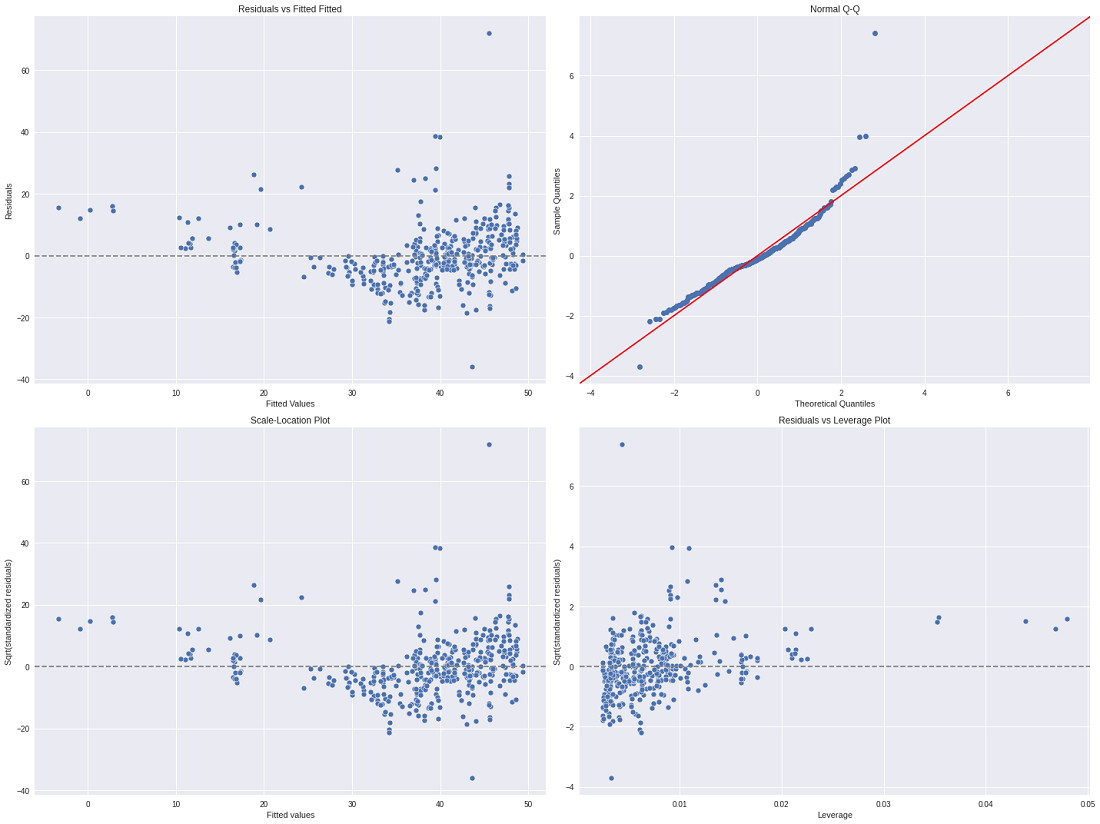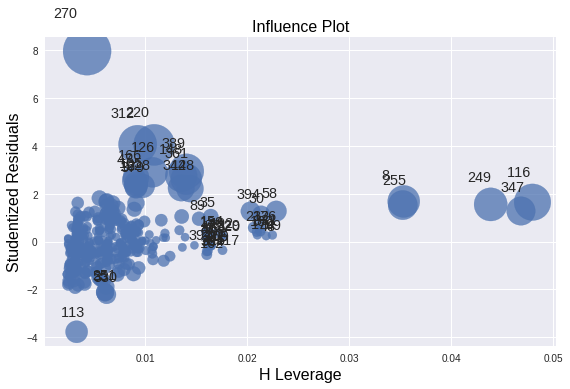# Residual Leverage Plot (Regression Diagnostic)

• Last Updated : 21 Sep, 2021

In linear or multiple regression, it is not enough to just fit the model into the dataset. But, it may not give the desired result. To apply the linear or multiple regression efficiently to the dataset. There are some assumptions that we need to check on the dataset that made linear/multiple regression efficient and generate better accuracy.

#### Assumptions of Regression

Regression analysis requires some assumptions to be followed by the dataset. These assumptions are:

Attention reader! Don’t stop learning now. Get hold of all the important Machine Learning Concepts with the Machine Learning Foundation Course at a student-friendly price and become industry ready.

• Observations are independent of each other. It should be correlated to another observation.
• Data is normally distributed.
• The relationship b/w the independent variable and the mean of the dependent variable is linear.
• The data is in homoscedasticity, which means the variance of the residual is the same for each value of the dependent variable.

To perform a good linear regression analysis, we also need to check whether these assumptions are violated:

• If the data contain non-linear trends then it will not be properly fitted by linear regression resulting in a high residual or error rate.
• To check for the normality in the dataset, draw a Q-Q plot on the data.
• The presence of correlation between observations is known as autocorrelation. We can check for the autocorrelation plot.
• The presence of homoscedasticity can be estimated with the plots such as the Scale Location plot, and the Residual vs Legacy plot.

### Regression Diagnostic Plots

The above plots can be used to validate and test the above assumptions are part of Regression Diagnostic. This diagnostic can be used to check whether the assumptions. Before we discuss the diagnostic plot one by one let’s discuss some important terms:

• Outliers: Outliers are the points that are distinct and deviant from the bulk of the dataset. In general, the outliers have high residual values means that the difference is greater than the b/w observed and predicted value.
• Leverage Points: A leverage point is defined as an observation that has a value of x that is far away from the mean of x.
• Influential Points: An influential observation is defined as an observation that has a large influence on the fit of the model. One method to find influential points is to compare the fit of the model with and without each observation.

Below are the plots that we used in the diagnostic plot:

• Residual vs fitted plot: The residual can be calculated as:This plot is used to check for linearity and homoscedasticity, if the model meets the condition of linear relationship then it should have a horizontal line with much deviation. If the model meets the condition for homoscedasticity, the graph should be equally spread around the y=0 line.

• Q-Q plot: This plot is used to check for the normality of the dataset, if there is normality that exists in the dataset then, the scatter points will be distributed along the 45 degrees dashed line.
• Scale-Location plot: It is a plot of square rooted standardized value vs predicted value. This plot is used for checking the homoscedasticity of residuals. Equally spread residuals across the horizontal line indicate the homoscedasticity of residuals.
• Residual vs Leverage plot/ Cook’s distance plot: The 4th point is the cook’s distance plot, which is used to measure the influence of the different plots. The Cook’s distance statistic for every observation measures the extent of change in model estimates when that particular observation is omitted. Cook distance plot the cook distance measure of each observation. whereas, Residual vs Leverage plot is the plot between standardized residuals and leverage points of the points.

### Implementation

In this implementation, we will be plotting different diagnostic plots. For that, we use the Real-Estate dataset and apply the Ordinary Least Square (OLS) Regression. We then plot the regression diagnostic plot and Cook distance plot.

## Python3

 `# imports``import` `numpy as np``import` `pandas as pd``import` `matplotlib.pyplot as plt``import` `statsmodels.api as sm``import` `statsmodels.formula.api as smf`` ` `# Load Real State Data``data ``=` `pd.read_csv(``'/content/Real estate.csv'``)``data.head()`` ` `# Fit a OLS regression variable``model ``=``smf.ols(formula``=``' Y ~ X3 + X2'``, data``=` `data )``results ``=` `model.fit()``print``(results.summary())`` ` `# Get different Variables for diagnostic``residuals ``=` `results.resid``fitted_value ``=` `results.fittedvalues``stand_resids ``=` `results.resid_pearson``influence ``=` `results.get_influence()``leverage ``=` `influence.hat_matrix_diag`` ` `# PLot different diagnostic plots``plt.rcParams[``"figure.figsize"``] ``=` `(``20``,``15``)``fig, ax ``=` `plt.subplots(nrows``=``2``, ncols``=``2``)`` ` `plt.style.use(``'seaborn'``)`` ` `# Residual vs Fitted Plot``sns.scatterplot(x``=``fitted_value, y``=``residuals, ax``=``ax[``0``, ``0``])``ax[``0``, ``0``].axhline(y``=``0``, color``=``'grey'``, linestyle``=``'dashed'``)``ax[``0``, ``0``].set_xlabel(``'Fitted Values'``)``ax[``0``, ``0``].set_ylabel(``'Residuals'``)``ax[``0``, ``0``].set_title(``'Residuals vs Fitted Fitted'``)`` ` `# Normal Q-Q plot``sm.qqplot(residuals, fit``=``True``, line``=``'45'``,ax``=``ax[``0``, ``1``], c``=``'#4C72B0'``)``ax[``0``, ``1``].set_title(``'Normal Q-Q'``)`` ` `# Scale-Location Plot``sns.scatterplot(x``=``fitted_value, y``=``residuals, ax``=``ax[``1``, ``0``])``ax[``1``, ``0``].axhline(y``=``0``, color``=``'grey'``, linestyle``=``'dashed'``)``ax[``1``, ``0``].set_xlabel(``'Fitted values'``)``ax[``1``, ``0``].set_ylabel(``'Sqrt(standardized residuals)'``)``ax[``1``, ``0``].set_title(``'Scale-Location Plot'``)`` ` `# Residual vs Leverage Plot``sns.scatterplot(x``=``leverage, y``=``stand_resids, ax``=``ax[``1``, ``1``])``ax[``1``, ``1``].axhline(y``=``0``, color``=``'grey'``, linestyle``=``'dashed'``)``ax[``1``, ``1``].set_xlabel(``'Leverage'``)``ax[``1``, ``1``].set_ylabel(``'Sqrt(standardized residuals)'``)``ax[``1``, ``1``].set_title(``'Residuals vs Leverage Plot'``)`` ` ` ` `plt.tight_layout()``plt.show()`` ` `# PLot Cook's distance plot``sm.graphics.influence_plot(results, criterion``=``"cooks"``)`
```------------
# data
No    X1    X2    X3    X4    X5    X6    Y
0    1    2012.917    32.0    84.87882    10    24.98298    121.54024    37.9
1    2    2012.917    19.5    306.59470    9    24.98034    121.53951    42.2
2    3    2013.583    13.3    561.98450    5    24.98746    121.54391    47.3
3    4    2013.500    13.3    561.98450    5    24.98746    121.54391    54.8
4    5    2012.833    5.0    390.56840    5    24.97937    121.54245    43.1
------------
OLS Regression Results
==============================================================================
Dep. Variable:                      Y   R-squared:                       0.491
Method:                 Least Squares   F-statistic:                     198.3
Date:                Thu, 19 Aug 2021   Prob (F-statistic):           5.07e-61
Time:                        17:56:17   Log-Likelihood:                -1527.9
No. Observations:                 414   AIC:                             3062.
Df Residuals:                     411   BIC:                             3074.
Df Model:                           2
Covariance Type:            nonrobust
==============================================================================
coef    std err          t      P>|t|      [0.025      0.975]
------------------------------------------------------------------------------
Intercept     49.8856      0.968     51.547      0.000      47.983      51.788
X3            -0.0072      0.000    -18.997      0.000      -0.008      -0.006
X2            -0.2310      0.042     -5.496      0.000      -0.314      -0.148
==============================================================================
Omnibus:                      161.397   Durbin-Watson:                   2.130
Prob(Omnibus):                  0.000   Jarque-Bera (JB):             1297.792
Skew:                           1.443   Prob(JB):                    1.54e-282
Kurtosis:                      11.180   Cond. No.                     3.37e+03
==============================================================================

Warnings:
 Standard Errors assume that the covariance matrix of the errors is correctly specified.
 The condition number is large, 3.37e+03. This might indicate that there are
strong multicollinearity or other numerical problems.
--------------```Regression Diagnostic PlotsCook distance Plot

### References:

My Personal Notes arrow_drop_up